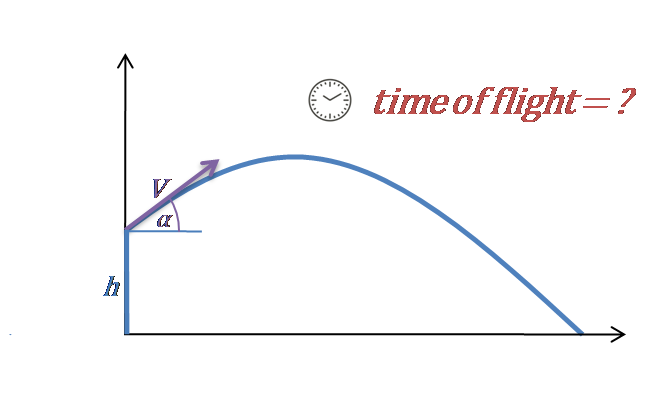# Time of Flight Calculator - Projectile Motion

Created by Hanna Pamuła, PhD candidate
Reviewed by Bogna Szyk
Last updated: May 20, 2020

With this time of flight calculator, you can easily calculate the time for which the projectile remains in the air. To solve this special case of projectile motion, all you need to enter are the initial velocity, angle, and height. Read on to find out more about the time of flight equations or simply play with the calculator instead!

## Time of flight equation

To define the time of flight equation, we should split the formulas into two cases:

1. Launching projectile from the ground (initial height = 0)

Let's start from an equation of motion:

y = V₀ * t * sin(α) - g * t² / 2

When the projectile hits the ground, the flight ends (y = 0).

0 = V₀ * t * sin(α) - g * t² / 2

Then, t is the time of flight - total time of the whole journey:

t = 2 * V₀ * sin(α) / g

Note that the air resistance is neglected.

Which angle of launch causes the projectile to spend the most time in the air? Let's have a look at the final time of flight equation again: the higher the value of a sine is, the longer the time in the air. The maximum value of sine occurs when angle = 90°. So if you throw an object upwards, it will keep moving for the longest time.

Additionally, if the velocity is equal to 0, then it's the case of a free fall.

2. Launching projectile from some height (so initial height > 0)

However, if the projectile is thrown from some elevation h, the formula is a bit more complicated:

t = [V₀ * sin(α) + √((V₀ * sin(α))² + 2 * g * h)] / g

## Time of flight exemplary calculations

Let's use this time of flight calculator to find out how long it takes for a pebble thrown from the edge of the Grand Canyon to hit the ground.

1. Type in the velocity value. Assume it's 16 ft/s.
2. Enter the angle. For example, 20°. If you choose angle = 0°, then it would be the example of horizontal projectile motion.
3. Finally, type in the initial height. Let's take the deepest point of the Canyon. It's 6,000 ft difference - over a mile! Type this value into our tool.
4. Time of flight calculator will show you the total time the pebble remains in the air. It's 19.5 seconds for our example.

All the calculations are made without taking into account air resistance. In reality, the trajectory would differ from the ideal parabola and the range would be shorter than the result obtained from the calculations.

Hanna Pamuła, PhD candidate
Velocity
ft/s
Angle of launch
deg
Initial height
ft
Time of flight
Time of flight
secPeople also viewed…

Addiction calculator tells you how much shorter your life would be if you were addicted to alcohol, cigarettes, cocaine, methamphetamine, methadone, or heroin.

### Brinell hardness number

Determine the indentation hardness of a material using the Brinell hardness number calculator.

### Free fall

Our free fall calculator can find the velocity of a falling object and the height it drops from.

### Wien's law

Check out Wien's law calculator to find the temperature of any hot object, basing on its thermal emission spectrum.